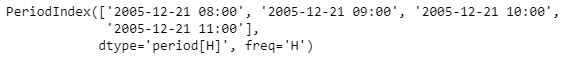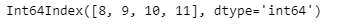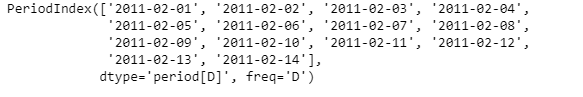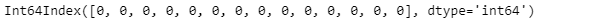Open in App
Not now

# Python | Pandas PeriodIndex.hour

• Last Updated : 25 Jan, 2023

Python is a great language for doing data analysis, primarily because of the fantastic ecosystem of data-centric python packages. Pandas is one of those packages and makes importing and analyzing data much easier. Pandas PeriodIndex.hour attribute return the hour of the period for the given PeriodIndex object.

Syntax : PeriodIndex.hour Parameters : None Return : Index object containing hour values.

Example #1: Use PeriodIndex.hour attribute to find the hour value of the period for the given PeriodIndex object.

## Python3

 `# importing pandas as pd``import` `pandas as pd` `# Create the PeriodIndex object``pidx ``=` `pd.PeriodIndex(start ``=``'2005-12-21 08:45 '``,``              ``end ``=``'2005-12-21 11:55'``, freq ``=``'H'``)` `# Print the PeriodIndex object``print``(pidx)`

Output :Now we will use the PeriodIndex.hour attribute to find the hour of the period in the given object.

## Python3

 `# return the hour value``pidx.hour`

Output :As we can see in the output, the PeriodIndex.hour attribute has returned an index object containing the hour values for each element of the given PeriodIndex object.   Example #2: Use PeriodIndex.hour attribute to find the hour of the period for the given PeriodIndex object.

## Python3

 `# importing pandas as pd``import` `pandas as pd` `# Create the PeriodIndex object``pidx ``=` `pd.PeriodIndex(start ``=``'2011-02-1'``,``           ``end ``=``'2011-02-14'``, freq ``=``'D'``)` `# Print the PeriodIndex object``print``(pidx)`

Output :Now we will use the PeriodIndex.hour attribute to find the hour value of the period in the given object.

## Python3

 `# return the hour value``pidx.hour`

Output :As we can see in the output, the PeriodIndex.hour attribute has returned an index object containing the hour values for each element of the given PeriodIndex object. As we can see the for the pidx object that we have applied daily frequency. So, the hour value is not set and hence the function has returned 0 for all the elements.

My Personal Notes arrow_drop_up